## Introduction

This package provides an easy way to indicate if two groups are significantly different. Commonly this is shown by a bar on top connecting the groups of interest which itself is annotated with the level of significance (NS, *, **, ***). The package provides a single layer (geom_signif) that takes the groups for comparison and the test (t.test, wilcox etc.) and adds the annotation to the plot.

## Citation

If you wish to cite this package in a publication, you can run the following command in your R console:

citation("ggsignif")
#>
#> To cite 'ggsignif' in publications use:
#>
#>   Ahlmann-Eltze, C., & Patil, I. (2021). ggsignif: R Package for
#>   Displaying Significance Brackets for 'ggplot2'. PsyArxiv.
#>   doi:10.31234/osf.io/7awm6
#>
#> A BibTeX entry for LaTeX users is
#>
#>   @Article{,
#>     title = {{ggsignif}: R Package for Displaying Significance Brackets for {'ggplot2'}},
#>     author = {Ahlmann-Eltze Constantin and Indrajeet Patil},
#>     year = {2021},
#>     journal = {PsyArxiv},
#>     url = {https://psyarxiv.com/7awm6},
#>     doi = {10.31234/osf.io/7awm6},
#>   }

## Example

You can first install this package from CRAN:

install.packages("ggsignif")

Or get the latest development version:

install.packages("remotes")
remotes::install_github("const-ae/ggsignif")

Plot significance

library(ggplot2)
library(ggsignif)

p1 <- ggplot(mpg, aes(class, hwy)) +
geom_boxplot() +
geom_signif(
comparisons = list(c("compact", "midsize"), c("minivan", "suv")),
map_signif_level = TRUE, textsize = 6
) +
ylim(NA, 48)
p1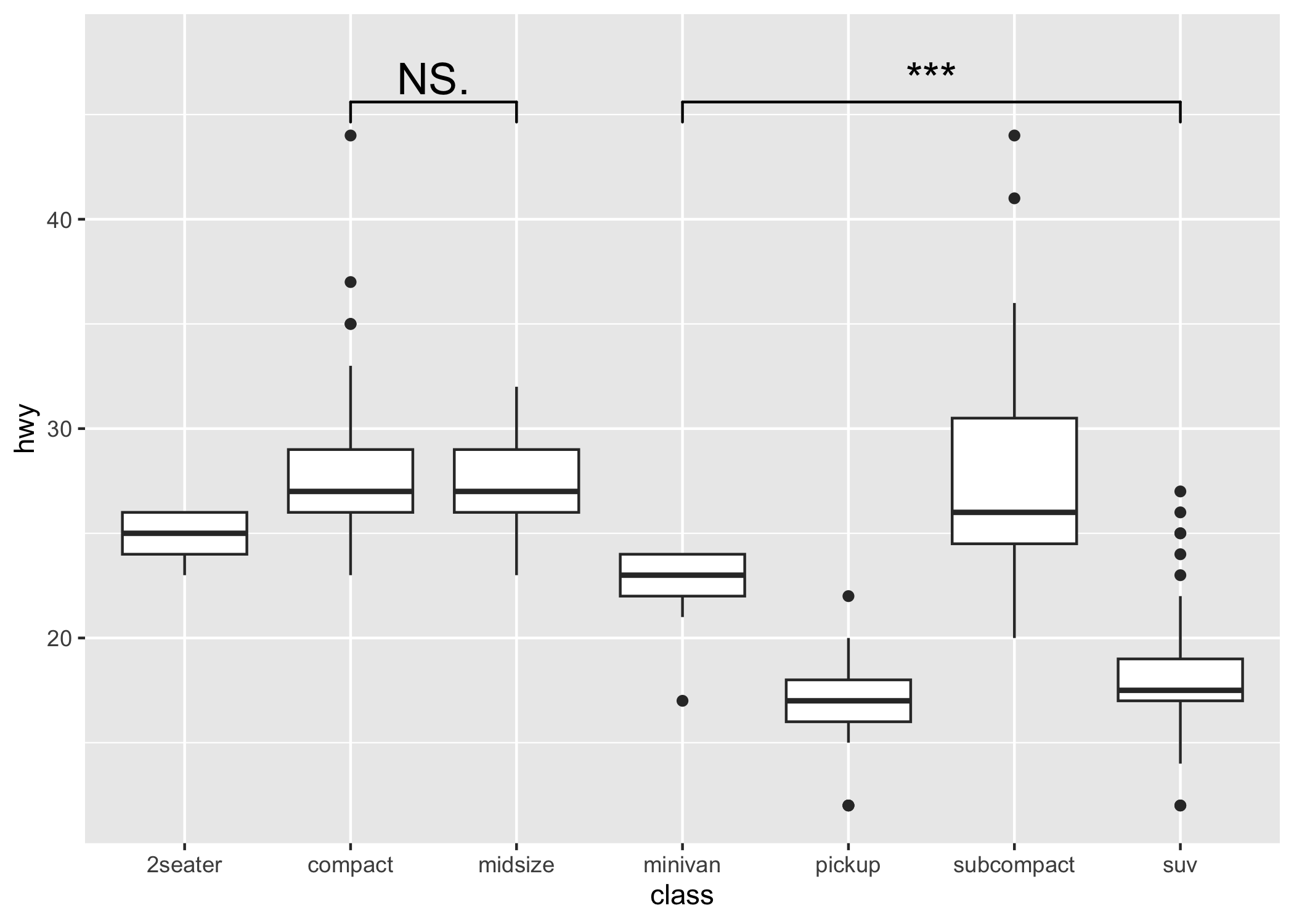Control the direction (either x or y) via orientation

p2 <- ggplot(
data = mpg,
mapping = aes(
x = hwy,
y = class
)
) +
geom_boxplot(
orientation = "y"
) +
geom_signif(
comparisons = list(
c("compact", "midsize"),
c("minivan", "suv")
),
map_signif_level = TRUE,
textsize = 6,
margin_top = 0.08,
step_increase = 0.05,
tip_length = 0.01,
orientation = "y"
)
p2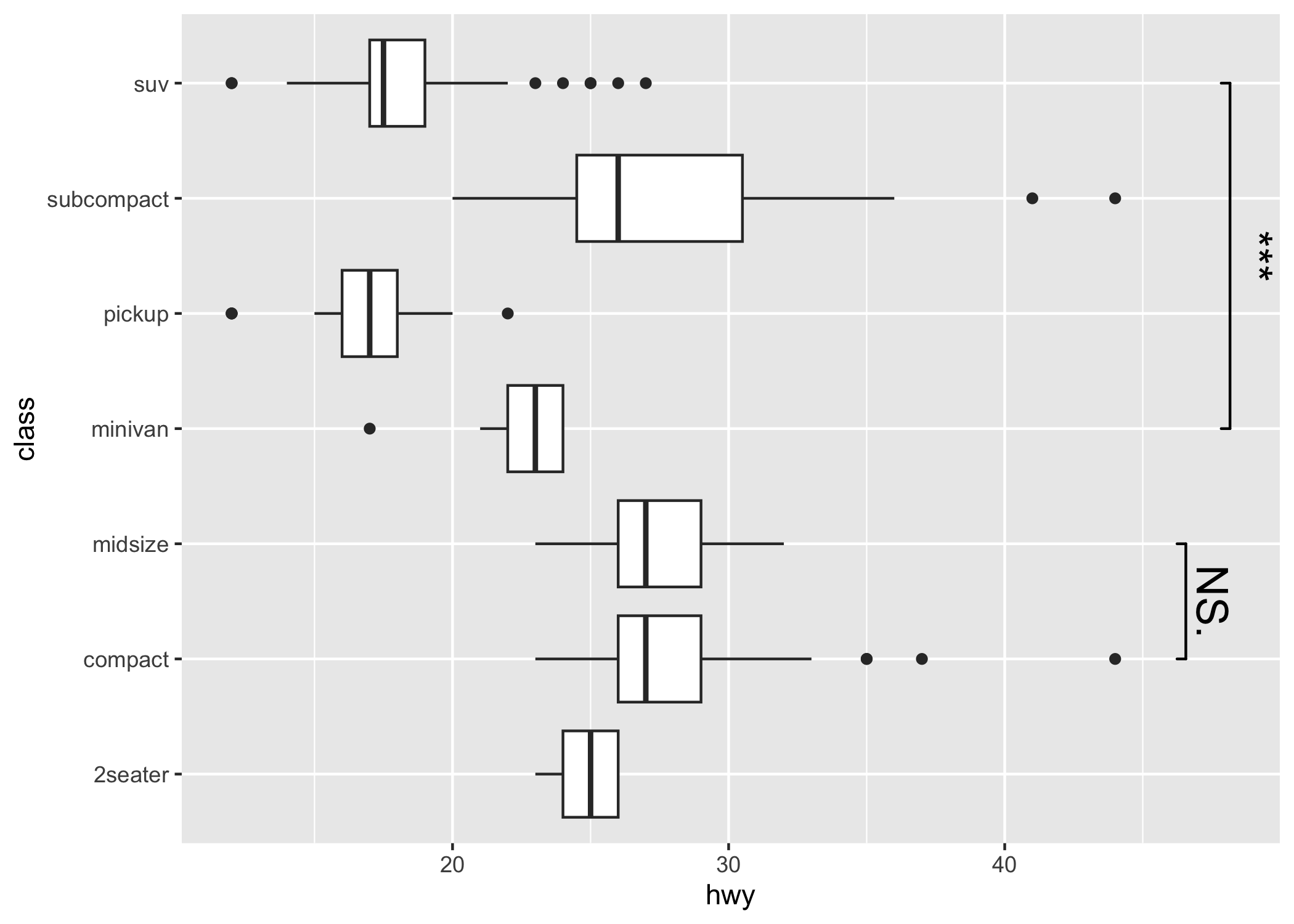Compatible with coord_flip

p1 + coord_flip()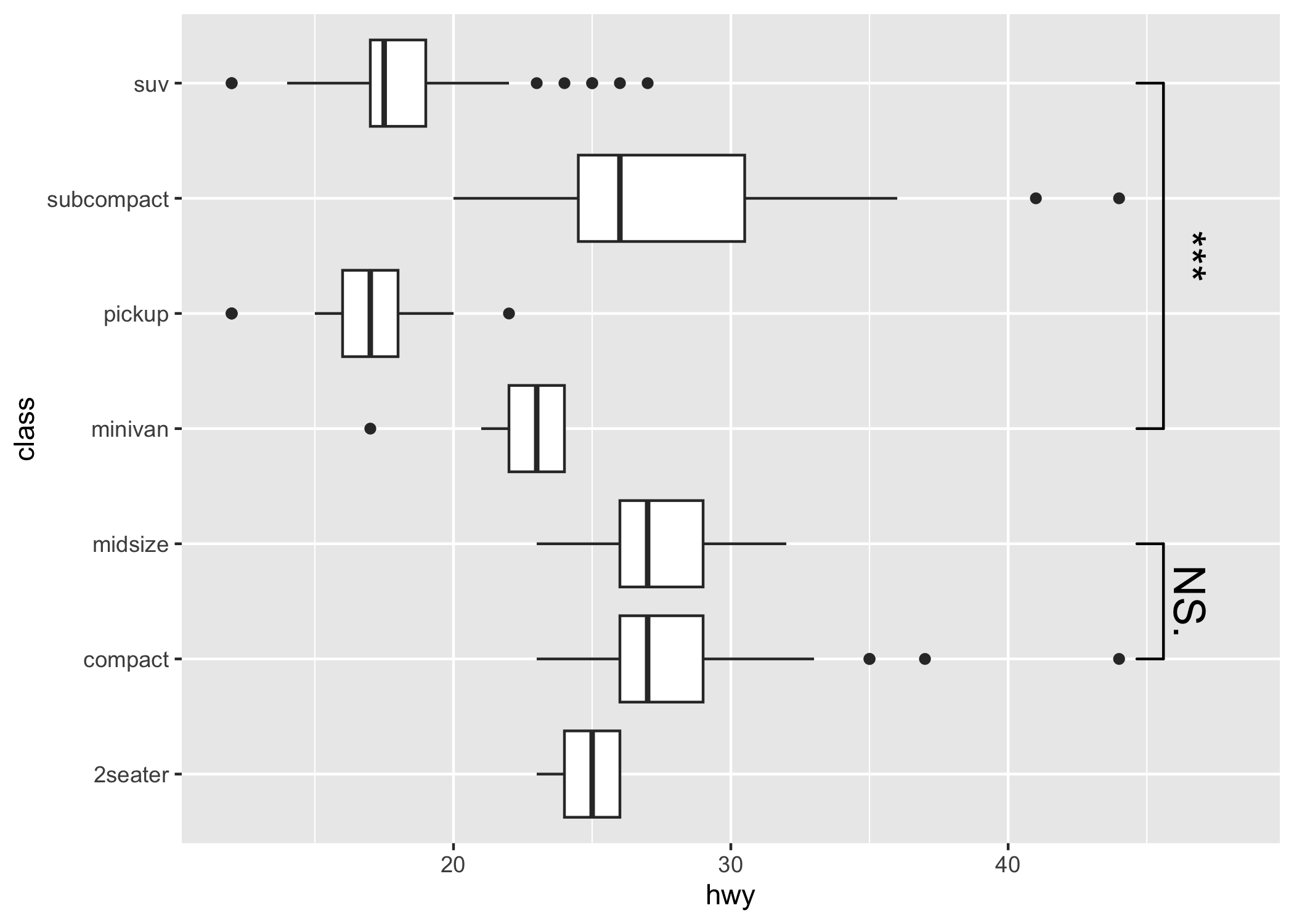Setting the precise location

This is important if you use position="dodge", because in that case I cannot calculate the correct position of the bars automatically.

# Calculate annotation
anno <- t.test(
iris[iris$Petal.Width > 1 & iris$Species == "versicolor", "Sepal.Width"],
iris[iris$Species == "virginica", "Sepal.Width"] )$p.value

# Make plot with custom x and y position of the bracket
ggplot(iris, aes(x = Species, y = Sepal.Width, fill = Petal.Width > 1)) +
geom_boxplot(position = "dodge") +
geom_signif(
annotation = formatC(anno, digits = 1),
y_position = 4.05, xmin = 2.2, xmax = 3,
tip_length = c(0.2, 0.04)
)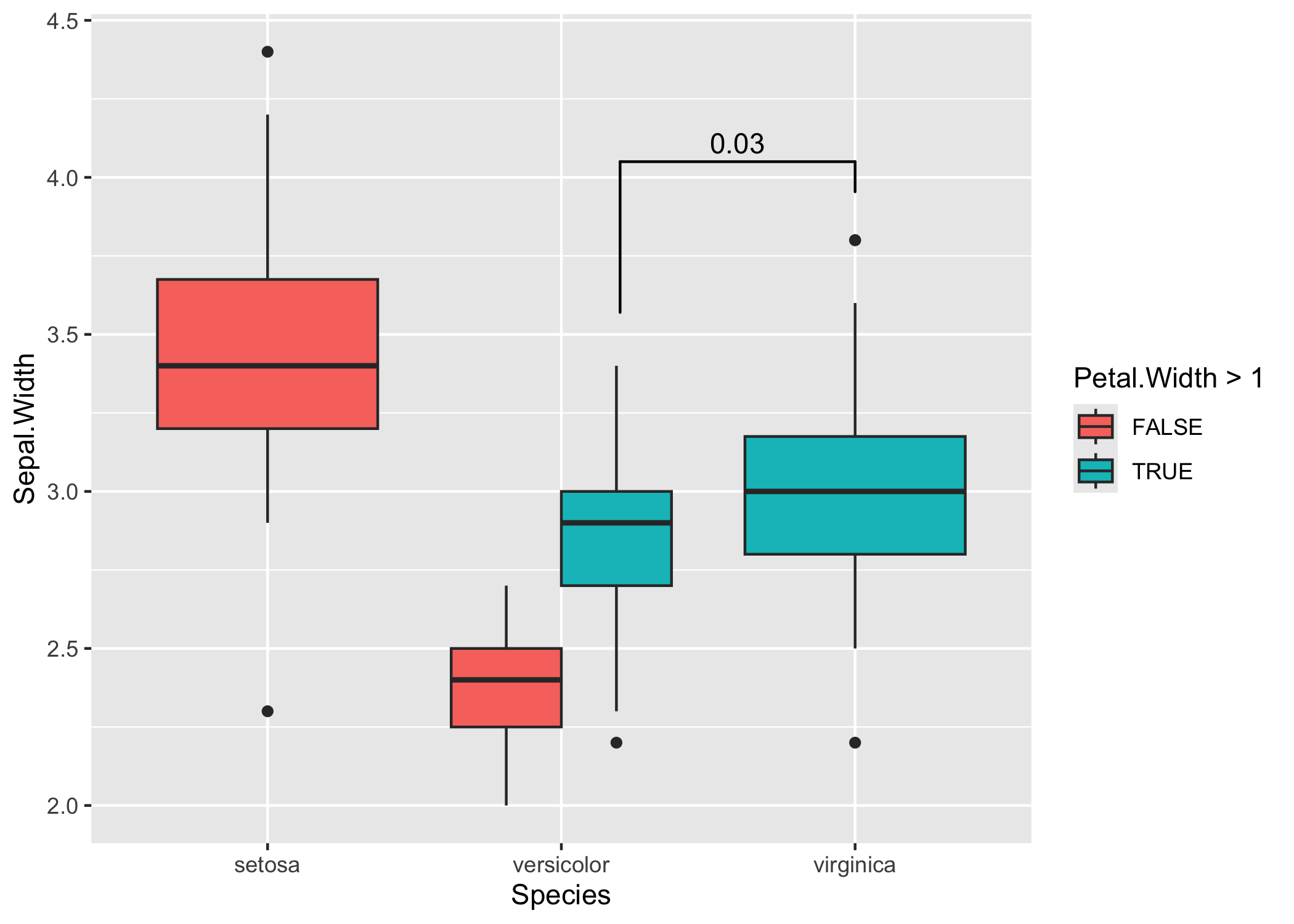Sometimes one needs to have a very fine tuned ability to set the location of the the significance bars in combination with facet_wrap or facet_grid. In those cases it you can set the flag manual=TRUE and provide the annotations as a data.frame:

annotation_df <- data.frame(
color = c("E", "H"),
start = c("Good", "Fair"),
end = c("Very Good", "Good"),
y = c(3.6, 4.7),
label = c("Comp. 1", "Comp. 2")
)

annotation_df
#>   color start       end   y   label
#> 1     E  Good Very Good 3.6 Comp. 1
#> 2     H  Fair      Good 4.7 Comp. 2

ggplot(diamonds, aes(x = cut, y = carat)) +
geom_boxplot() +
geom_signif(
data = annotation_df,
aes(xmin = start, xmax = end, annotations = label, y_position = y),
textsize = 3, vjust = -0.2,
manual = TRUE
) +
facet_wrap(~color) +
ylim(NA, 5.3)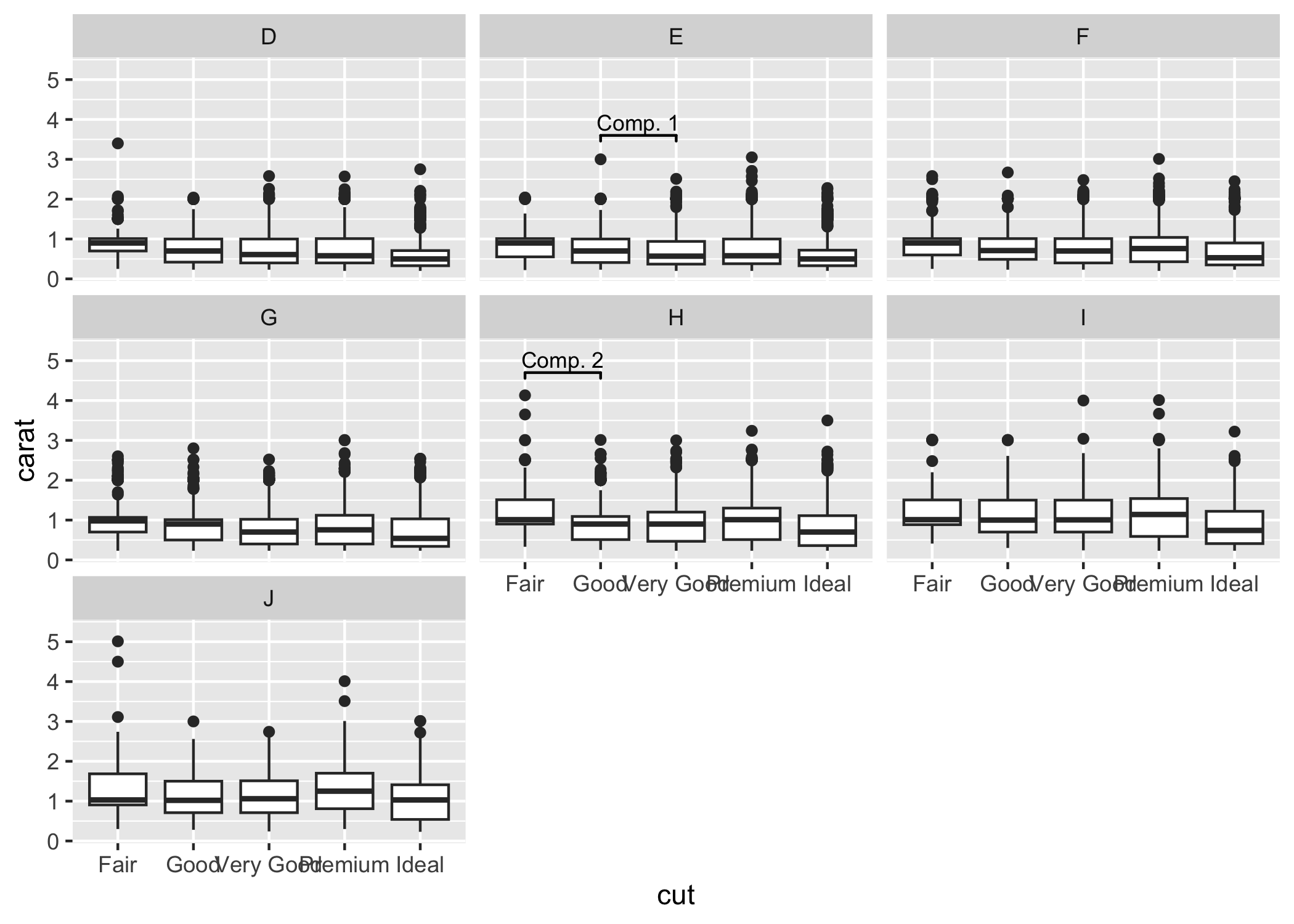You can ignore the warning about the missing aesthetics.

For further details, see: https://const-ae.github.io/ggsignif/articles/intro.html

## Maintenance

This package is provided as is and we currently don’t have any plans and the capacity to add any new features to it. If there is nonetheless a feature which you would like to see in the package, you are always welcome to submit pull request, which we will try to address as soon as possible.

## Code of Conduct

Please note that the ggsignif project is released with a Contributor Code of Conduct. By contributing to this project, you agree to abide by its terms.